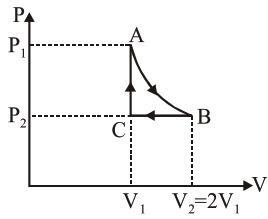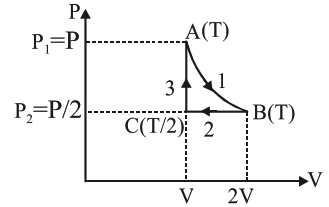Deepak Scored 45->99%ile with Bounce Back Crack Course. You can do it too!

# n mole a perfect gas

Question:

$\mathrm{n}$ mole a perfect gas undergoes a cyclic process ABCA (see figure) consisting of the following processes.

$\mathrm{A} \rightarrow \mathrm{B}:$ Isothermal expansion at temperature $T$ so that the volume is doubled from $V_{1}$ to $V_{2}=2 V_{1}$ and pressure changes from $P_{1}$ to $P_{2}$.

$\mathrm{B} \rightarrow \mathrm{C}:$ Isobaric compression at pressure $\mathrm{P}_{2}$ to initial volume $V_{1}$.

$\mathrm{C} \rightarrow \mathrm{A}:$ Isochoric change leading to change of pressure from $P_{2}$ to $P_{1}$.

Total workdone in the complete cycle ABCA is :1. 0

2. $\mathrm{nRT}\left(\ln 2+\frac{1}{2}\right)$

3. $\mathrm{nRT} \ln 2$

4. $\mathrm{nRT}\left(\ln 2-\frac{1}{2}\right)$

Correct Option: , 4

Solution:

$\mathrm{W}_{\text {Isothermal }}=\mathrm{nRTln}\left(\frac{\mathrm{v}_{2}}{\mathrm{v}_{1}}\right)$

$\mathrm{W}_{\text {Isobaric }}=\mathrm{P} \Delta \mathrm{V}=\mathrm{nR} \Delta \mathrm{T}$

$\mathrm{W}_{\text {Isochoric }}=0$$\mathrm{W}_{1}=\mathrm{nRT} \ln \left(\frac{2 \mathrm{~V}}{\mathrm{~V}}\right)=\mathrm{nRT} \ln 2$

$\mathrm{W}_{2}=\mathrm{nR}\left(\frac{\mathrm{T}}{2}-\mathrm{T}\right)=-\mathrm{nR} \frac{\mathrm{T}}{2}$

$\mathrm{W}_{3}=0$

$\Rightarrow \mathrm{W}_{\text {net }}=\mathrm{W}_{1}+\mathrm{W}_{2}+\mathrm{W}_{3}$

$\mathrm{W}_{\mathrm{net}}=\mathrm{nRT}\left(\ln 2-\frac{1}{2}\right)$